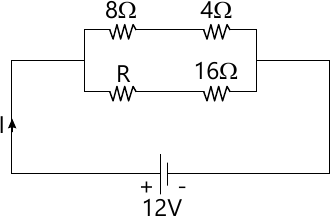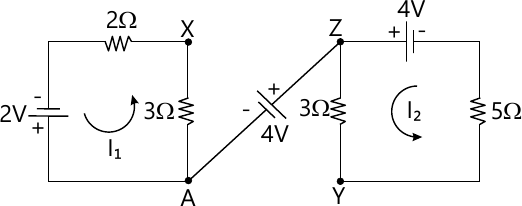MORE IN Basic Electrical Engg.
VTU First Year Engineering (P Cycle) (Semester 1)
Basic Electrical Engg.
June 2015
Total marks: --
Total time: --
INSTRUCTIONS
(1) Assume appropriate data and state your reasons
(2) Marks are given to the right of every question
(3) Draw neat diagrams wherever necessary

1 (a) State and explain Faraday's laws of electromagnetic induction
6 M
1 (b) An air cored solenoid has a length of 50 cm and a diameter of 2 cm. Calculate its inductances if it has 1000 turns and also find the energy stored in it current rises from zero to 50A
6 M
1 (c) If the total power displayed in the circuit shown is 18W, find the value of 'R' and its current8 M

2 (a) State the following
i) Fleming right hand rule
ii) Fleming left hand rule
6 M
2 (b) A closed iron of mean diameter 12 cm made from round iron bar of diameter 2 cm. It has a uniform winding of 1000 turns. Calculate the current required to produce a flux density of 1.5 Wb/m2 given that relative permeability is 1250. Hence calculate the self inductance
8 M
2 (c) What is the potential difference between the point x and y in the network shown ?6 M

3 (a) Explain the characteristics of DC series motor with a neat diagram
6 M
3 (b) Explain the significance of back emf in DC motor
5 M
3 (c) 4 pole DC shunt motor takes 22.5A from a 250V supply, Ra=0.5Ω and Rsh=125 ω. The armature is wave wound with 300 conductors. If the flux per pole is 0.02 Wb. Calculate
i) speed
ii) torque developed
iii) power developed
9 M

4 (a) With a neat diagram explain the construction and working of dynamo-meter type wattmeter.
6 M
4 (b) 4 pole generator with wave wound armature has 51 slots, each having 24 conductors. The flux per ople is 0.01 Wb. At what speed must the armature rotate to give an induced emf of 220V? What will be the voltage developed if the winding is lap and the armature rotates at the same speed.
10 M
4 (c) Explain with diagram , the construction features of various parts of a DC generator
4 M

5 (a) What is meant by power in AC circuit? What is its significance in AC circuits
6 M
5 (b) Draw and explain the wiring diagram for the 3- way control of lamp
6 M
5 (c) A series circuit with resistance of 10 Ω, inductance of 0.2H and capacitance of 40 μF is supplied with a 100 V supply at 50Hz. Find the current, power and power factor of the circuit
8 M

6 (a) State from factor of an alternating quantity. Derive the expression for it
8 M
6 (b) Show that the average power consumed in a pure capacitance is zero. Draw the neat waveform for voltage, current , power
6 M
6 (c) With a neat diagram , explain explain pipe earthing
6 M

7 (a) With the usual notation, derive the expression for EMF equation of an alternator
6 M
7 (b) Establish the relationship between phase and line values of voltage and current in 3 phase delta connected circuit. Show the phasor diagram neatly.
6 M
7 (c) A balanced star connected load of (8+6J) Ω/phase is connected to 3 phase , 230V supply. Find the line current, power factor power reactive volt ampere and total volt ampere
8 M

8 (a) Show that the power in balanced 3 phase circuit can be measured by 2 wattmeters. Draw the circuit and vector diagram
8 M
8 (b) Explain the generation of 2 phase AC voltage
4 M
8 (c) A 3 phase 50 Hz 16 pole generator with star connected winding has 144 slots with conductor /slot is 10. the flux per pole is 24.8m Wb is sinusoidally distributed. The coils are full pitched. Find i) Speed ii) the line emf
8 M

9 (a) Explain the construction and working principle of a transformer with a neat sketch
8 M
9 (b) Explain the concept of rotating magnetic field in a 3φ induction motor.
6 M
9 (c) The frequency of the emf in the stator of 4 pole induction motor is 50Hz and in the motor is 1.5HZ. What is the slip and at what speed is the motor running?
6 M

10 (a) What is 'slip' in an induction motor? Explain why slip is never zero in an induction motor
6 M
10 (b) A single phase transformer has 400 turns primary and 1000 secondary turns. The net cross- sectional area of the core is 60cm2. The primary winding is connected to a 500V, 50Hz supply. Find :
i) peak value of flux density
ii) emf induced in the secondary winding.
6 M
10 (c) The maximum efficiency at full load and unity p.f of a single phase 25KVA, 500/1000V, 50Hz transformer is 98%. determine its efficiency at
i) 75% load 0.9 p.f and